Next: Composition rules and KL-structures Up: KL-components Previous: Lift definitions

#### Decomposition methods

specify how a task is achieved through the execution of a set of subtasks, and regulate the data and control flows between such subtasks. Task decomposition methods are formally specified through QDL meta-theories, whose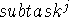predicate meta-variables describe the task decomposition they introduce. The number and syntactical structure of the arguments in each atomic formula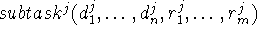describe the requirements on methods imposed by. The axioms and program parts respectively specify the data and control flow the method establishes among its subtasks. The description of the data flow a decomposition method establishes among its subtasks is done through the definition of input axioms, which specify how the contents of the state variables of some subtasks are used by other subtasks. The control flow among its subtasks is described by a QDL program, that specifies whichshould be invoked in each state of execution. The execution state of a decomposition method is described by the values of the state variables of all its subtasks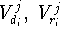.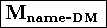sorts sort tree
constants (constantsymbol +: sortsymbol) *
functions (functionsymbol +: sortsymb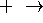sortsymbol) *
predicates (predicatesymbol +: sortsymbol *) *
variables (variablesymbol +: sortsymbol) *
metavariables (varsymbol +: metatype) *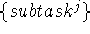: predicate
axioms (input axiom) *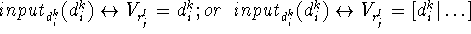program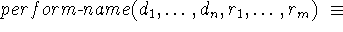a QDL program made from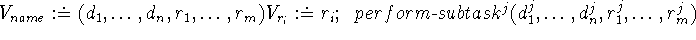conditions and programs on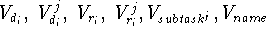The following example shows the formal description of a decomposition method.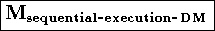predicates sequentialexecution
variables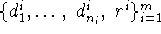metavariables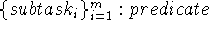axioms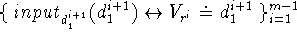program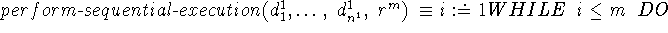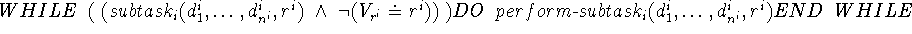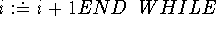jsierra@cs.stanford.edu